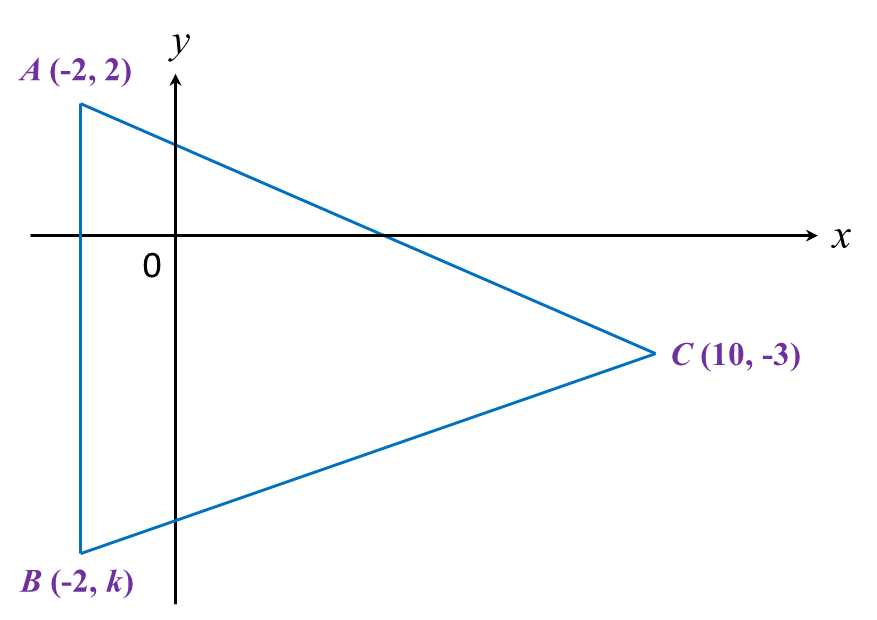# 5.2.4 Coordinates, PT3 Focus Practice

Question 9:
In diagram below, ABC is an isosceles triangle.Find
(a) the value of k,
(b) the length of BC.

Solution:

Question 10:
Diagram below shows a rhombus PQRS drawn on a Cartesian plane. PS is parallel to x-axis.Given the perimeter of PQRS is 40 units, find the coordinates of point R.

Solution: